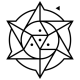## Post without words #24Associate Professor of Computer Science at Hendrix College. Functional programmer, mathematician, teacher, pianist, follower of Jesus.
This entry was posted in computation, number theory, posts without words, primes and tagged , , , . Bookmark the permalink.

### 5 Responses to Post without words #24

1.Denis says:

Clearly something multiplicative or factor-based. I think the blue lines are primes, with 2 and 3 at the top point. Wondering about the mostly-red line near the bottom.

•Brent says:

Right about primes. The mostly-red line is indeed very interesting.

2.blaisepascal2014 says:

By the structure (a triangle), it feels like it is the result of some function$f(i,j), j < i$. The blue lines look like they are when$i$ is prime.
Since the "words" (aka "tags") attached include Carmichael, Fermat, primality, and test, I'm going to guess that the colors correspond to values of$j^{i-1} \mod i$. Fermat's Little Theorem states that if$i$ is prime, then$j^{i-1} \equiv 1 \mod i$ for all$j$.
That would account for the blue lines (1) for each prime row. I would guess that the 561st row is red. 561 is the first Carmichael number, which is composite but "passes" Fermat's Little Theorem.

•Brent says:

Right, except the colors are more “logical” than numerical. I.e. the colors don’t correspond directly to the value of$j^{i-1} \bmod i$; there are four different colors that encode something about the pair$(i,j)$.